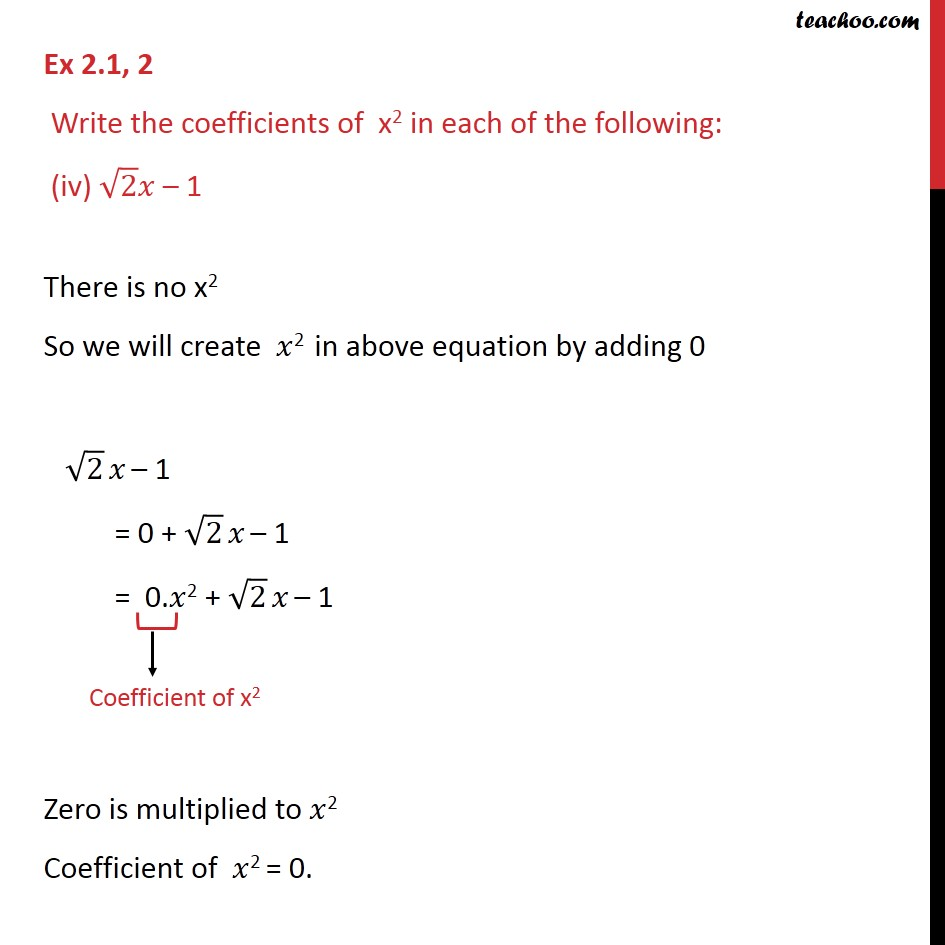Ex 2.1

Chapter 2 Class 9 Polynomials
Serial order wiseLearn in your speed, with individual attention - Teachoo Maths 1-on-1 Class

### Transcript

Ex 2.1, 2 Write the coefficients of x2 in each of the following: (iv) √2 𝑥 – 1 There is no x2 So we will create 𝑥2 in above equation by adding 0 √2 𝑥 – 1 = 0 + √2 𝑥 – 1 = 0.𝑥2 + √2 𝑥 – 1 Zero is multiplied to 𝑥2 Coefficient of 𝑥2 = 0.